# Category: featured

A nice interview with Jacques Roubaud (the guy responsible for Bourbaki’s death announcement) in the courtyard of the ENS. He talks about go, categories, the composition of his book $\in$ and, of course, Grothendieck and Bourbaki.

Clearly there are pop-math books like dedicated to $\pi$ or $e$, but I don’t know just one novel having as its title a single mathematical symbol : $\in$ by Jacques Roubaud, which appeared in 1967.

The book consists of 361 small texts, 180 for the white stones and 181 for the black stones in a game of go, between Masami Shinohara (8th dan) and Mitsuo Takei (2nd Kyu). Here’s the game:In the interview, Roubaud tells that go became quite popular in the mid sixties among French mathematicians, or at least those in the circle of Chevalley, who discovered the game in Japan and became a go-envangelist on his return to Paris.

In the preface to $\in$, the reader is invited to read it in a variety of possible ways. Either by paying attention to certain groupings of stones on the board, the corresponding texts sharing a common theme. Or, by reading them in order of how the go-game evolved (the numbering of white and black stones is not the same as the texts appearing in the book, fortunately there’s a conversion table on pages 153-155).

Or you can read them by paragraph, and each paragraph has as its title a mathematical symbol. We have $\in$, $\supset$, $\Box$, Hilbert’s $\tau$ and an imagined symbol ‘Symbole de la réflexion’, which are two mirrored and overlapping $\in$’s. For more information, thereader should consult the “Dictionnaire de la langue mathématique” by Lachatre and … Grothendieck.

According to the ‘bibliographie’ below it is number 17 in the ‘Publications of the L.I.T’.Other ‘odd’ books in the list are: Bourbaki’s book on set theory, the thesis of Jean Benabou (who is responsible for Roubaud’s conversion from solving the exercises in Bourbaki to doing work in category theory. Roubaud also claims in the interview that category theory inspired him in the composition of the book $\in$) and there’s also Guillaume d’Ockham’s ‘Summa logicae’…

In the spring of 2009 I did spend a fortnight dog-sitting in a huge house in the countryside, belonging to my parents-in-law, who both passed away the year before.

That particular day it was raining and thundering heavily. To distract myself from the sombre and spooky atmosphere in the house I began to surf the web looking for material for a new series of blogposts (yes, in those days I was still thinking in ‘series’ of posts…).

Bookmarks for that day tell me that the first picture grasping my attention was Salvador Dali’s Sacrament of the last supper, in particular the depicted partial dodecahedron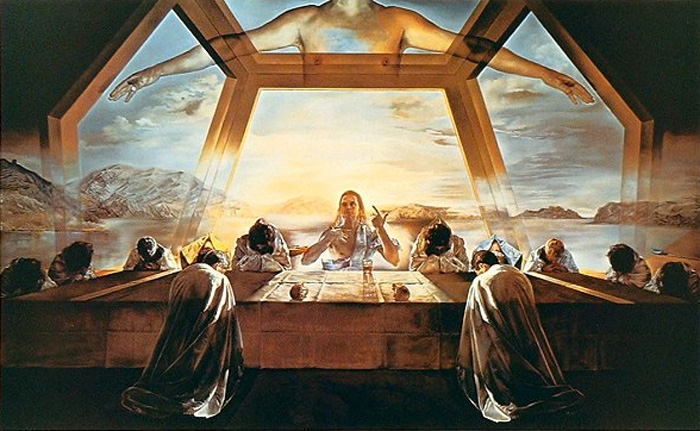I did compare it with Leonardo’s last supper and in the process stumbled upon Leonardo’s drawings of polyhedra, among which these two dodecahedra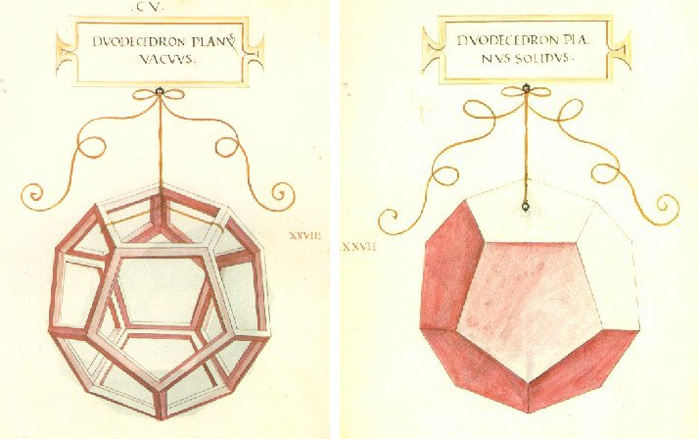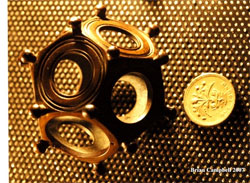From there it went on and on : the Mystery of the 2nd and 3rd Century Roman Dodecahedron and its posible use ‘casting dodecahedra’ in Tarot Divination Without Tarot Cards or as an astronomical instrument, a text on polyhedra and plagiarism in the renaissance, the history of the truncated icosahedron, a Bosnian pyramid and its stone balls, the sacred geometry of the dodecahedron, mathematics in the Vatican library, and on and on and on…

By noon, I felt I had enough material to post for a couple of weeks on “platonic solids through the ages”.

In between two rain showers, I walked the dog, had a quick lunch, and started writing.

I wanted to approach the topic in chronological order, and as I had done already a quicky on Scottish solids, the first post of the series would have to extend on this picture of five stone balls from the Ashmolean museum (or so it was claimed).

So, I hunted for extra pictures of these stone balls from the Ashmolean, and when comparing the two, clearly something had to be wrong…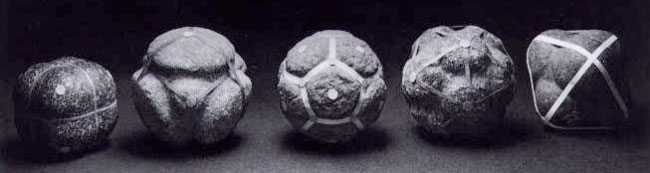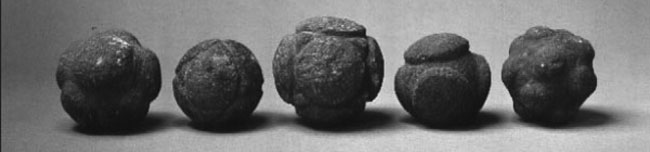It took me a couple of hours to catch up with the scientific literature on these Scottish balls, their cataloguing system and the museums of Scotland and England that house them.

Around 4pm I had compiled a list of all potential dodecahedra and icosahedra Scottish balls: ‘there are only 8 possible candidates for a Scottish dodecahedron (below their catalogue numbers, indicating to the knowledgeable which museum owns them and where they were found)

NMA AS 103 : Aberdeenshire
AS 109 : Aberdeenshire
AS 116 : Aberdeenshire (prob)
AUM 159/9 : Lambhill Farm, Fyvie, Aberdeenshire
Dundee : Dyce, Aberdeenshire
GAGM 55.96 : Aberdeenshire
Montrose = Cast NMA AS 26 : Freelands, Glasterlaw, Angus

The case for a Scottish icosahedron looks even worse. Only two balls have exactly 20 knobs

NMA AS 110 : Aberdeenshire
GAGM 92 106.1. : Countesswells, Aberdeenshire’

About an hour later I’d written the post, clicked the ‘Publish’ button and The Scottish solids hoax, began to live a life of its own!

From the numerous reactions let me single out 3 follow-ups which I believe to be most important.

John McKay and Tom Leinster did some legwork, tracking down resp. photographer and one of the 20 knobs balls.

John Baez gave a talk at an AMS meeting dedicated to the history of mathematics on Who discovered the icosahedron? mentioning my post and extending it by:

“And here is where I did a little research of my own. The library at UC Riverside has a copy of Keith Critchlow’s 1979 book Time Stands Still. In this book, we see the same photo of stones with ribbons that appears in Lawlor’s book – the photo that Atiyah and Suttcliffe use. In Critchlow’s book, these stones are called “a full set of Neolithic ‘Platonic solids'”. He says they were photographed by one Graham Challifour – but he gives no information as to where they came from!

And Critchlow explicitly denies that the Ashmolean has an icosahedral stone! He writes:

… the author has, during the day, handled five of these remarkable objects in the Ashmolean museum…. I was rapt in admiration as I turned over these remarkable stone objects when another was handed to me which I took to be an icosahedron…. On careful scrutiny, after establishing apparent fivefold symmetry on a number of the axes, a count-up of the projections revealed 14! So it was not an icosahedron.”

And now there is even a published paper out!

Bob Lloyd wrote How old are the Platonic solids?, published in BSHM Bulletin: Journal of the British Society for the History of Mathematics. The full article is behind a paywall but Bob graciously send me a copy.Bob believes the balls in the picture to belong to the Scottish ‘National Museum of Antiquities’ (NMA in the Marshall list), now the National Museum of Scotland (NMS) in Edinburgh.

He believes the third and fourth ball to be two pictures of the same object “recorded as having been discovered in Aberdeenshire” so it should be NMA AS 103 : Aberdeenshire in the above list. (Or, the other one may be NMA AS 26?).

He also attempts to identify the other 3 balls with objects in the NMS-collection. In short, he gives compelling evidence that the picture must have been taking in Edinburg and exists of genuine artifacts.

Perhaps even more important is that he finally puts the case of a Scottish icosahedron to rest. As mentioned above, there are just two candidates NMA AS 110 (Edinburg) and GAGM 92 106.1 (Glasgow). He writes:

“According to the Marshall list, there are only two balls known which have 20K; one of these is at the NMS. Alan Saville, Senior Curator for Earliest Prehistory at this Museum, has provided a photograph which shows that this object is complex, and certainly not a dodecahedron. It could be considered as a modified octahedron, with five large knobs in the usual positions, but with the sixth octahedral position occupied by twelve small knobs, and in addition there are also three small triangles carved at some of the interstices, the three-fold positions of the ‘octahedron’. These make up a total of twenty ‘protrusions’, though the word ‘knobs’ is hard to justify.

The other 20K object is at the Kelvingrove museum in Glasgow. Photographs taken by Tracey Hawkins, assistant curator, show that this also is very far from being a dodecahedron, though this time there are twenty clearly defined knobs of roughly the same size. The shape is somewhat irregular, but two six-sided pyramids can be picked out, and much of the structure, though not all, is deltahedral in form, with sets of three balls at the corners of equilateral triangles.”

So, sadly for John McKay, there is no Scottish icosahedron out there!

One final comment. Both John Baez (in a comment) and Bob Lloyd (in a comment and in his paper) argue that I shouldn’t have used the term “hoax” for something that is merely a ‘matter of sloppy scholarship’.

My apologies.

Given Bob’s evidence that the balls in the picture are genuine artifacts, I have deleted the ‘fabrication or falsification’-phrase in the original post.

Summarizing : the Challifour photograph is not taken at the Ashmolean museum, but at the National Museum of Scotland in Edinburgh and consists of 5 of their artifacts (or 4 if ball 3 and 4 are identical) vaguely resembling cube, tetrahedron, dodecahedron (twice) and octahedron. The fifth Platonic solid, the icosahedron, remains elusive.

Sometimes a MathOverflow question gets deleted before I can post a reply…

Yesterday (New-Year) PD1&2 were visiting, so I merely bookmarked the What is the knot associated to a prime?-topic, promising myself to reply to it this morning, only to find out that the page no longer exists.

From what I recall, the OP interpreted one of my slides of the April 1st-Alumni talk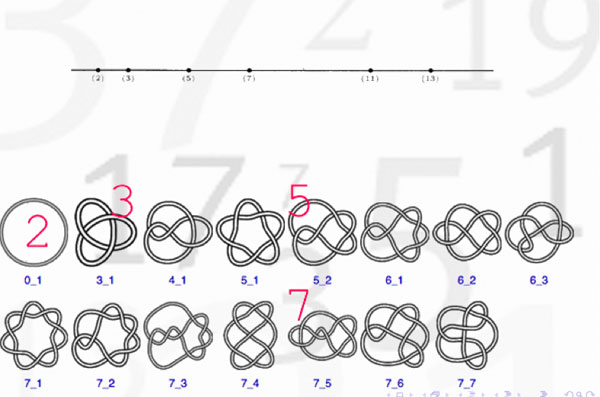as indicating that there might be a procedure to assign to a prime number a specific knot. Here’s the little I know about this :

Artin-Verdier duality in etale cohomology suggests that $Spec(\mathbb{Z})$ is a 3-dimensional manifold, as Barry Mazur pointed out in this paper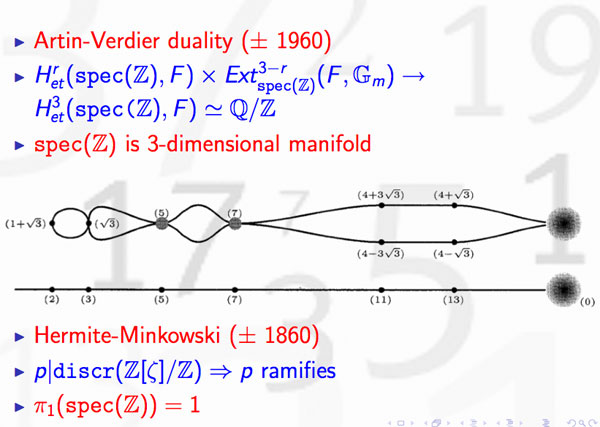The theory of discriminants shows that there are no non-trivial global etale extensions of $Spec(\mathbb{Z})$, whence its (algebraic) fundamental group should be trivial. By Poincare-Perelman this then implies that one should view $Spec(\mathbb{Z})$ as the three-sphere $S^3$. Note that there is no ambiguity in this direction. However, as there are other rings of integers in number fields having trivial fundamental group, the correspondence is not perfect.

Okay, but then primes should correspond to certain submanifolds of $S^3$ and as the algebraic fundamental group of $Spec(\mathbb{F}_p)$ is the profinite completion of $\mathbb{Z}$, the first option that comes to mind are circles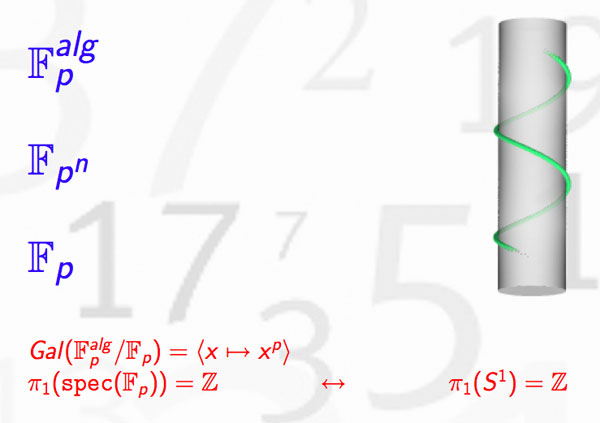Hence, primes might be viewed as circles embedded in $S^3$, that is, as knots! But which knots? Well, as far as I know, nobody has a procedure to assign a knot to a prime number, let alone one having p crossings. What is known, however, is that different primes must correspond to different knots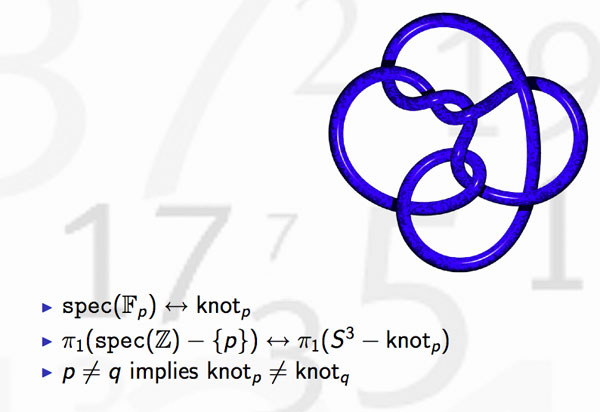because the algebraic fundamental groups of $Spec(\mathbb{Z})- { p }$ differ for distinct primes. This was the statement I wanted to illustrate in the first slide.

But, the story goes a lot further. Knots may be linked and one can detect this by calculating the link-number, which is symmetric in the two knots. In number theory, the Legendre symbol, plays a similar role thanks to quadratic reciprocity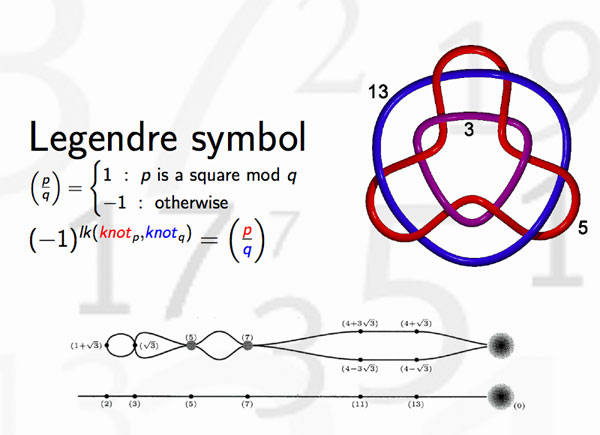and hence we can view the Legendre symbol as indicating whether the knots corresponding to different primes are linked or not. Whereas it is natural in knot theory to investigate whether collections of 3, 4 or 27 knots are intricately linked (or not), few people would consider the problem whether one collection of 27 primes differs from another set of 27 primes worthy of investigation.

There’s one noteworthy exception, the Redei symbol which we can now view as giving information about the link-behavior of the knots associated to three different primes. For example, one can hunt for prime-triples whose knots link as the Borromean rings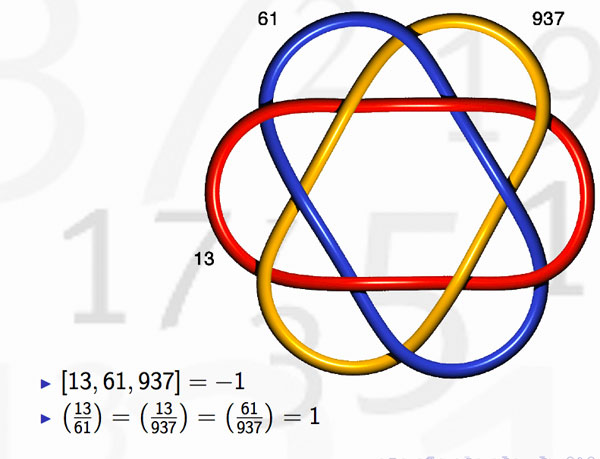(note that the knots corresponding to the three primes are not the unknot but more complicated). Here’s where the story gets interesting : in number-theory one would like to discover ‘higher reciprocity laws’ (for collections of n prime numbers) by imitating higher-link invariants in knot-theory. This should be done by trying to correspond filtrations on the fundamental group of the knot-complement to that of the algebraic fundamental group of $Spec(\mathbb{Z})-{ p }$ This project is called arithmetic topology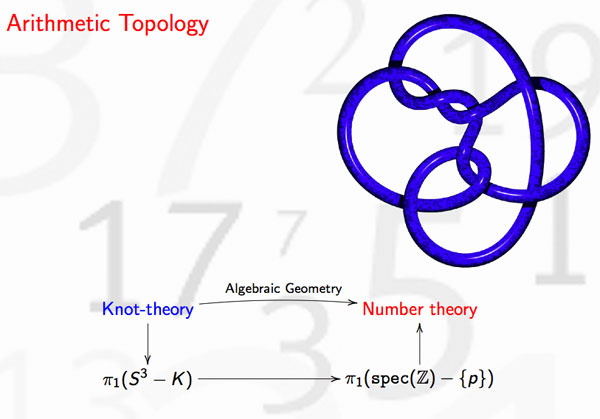Perhaps I should make a pod- or vod-cast of that 20 minute talk, one day…

Here’s a tiny problem illustrating our limited knowledge of finite fields : “Imagine an infinite queue of Knights ${ K_1,K_2,K_3,\ldots }$, waiting to be seated at the unit-circular table. The master of ceremony (that is, you) must give Knights $K_a$ and $K_b$ a place at an odd root of unity, say $\omega_a$ and $\omega_b$, such that the seat at the odd root of unity $\omega_a \times \omega_b$ must be given to the Knight $K_{a \otimes b}$, where $a \otimes b$ is the Nim-multiplication of $a$ and $b$. Which place would you offer to Knight $K_{16}$, or Knight $K_n$, or, if you’re into ordinals, Knight $K_{\omega}$?”

What does this have to do with finite fields? Well, consider the simplest of all finite field $\mathbb{F}_2 = { 0,1 }$ and consider its algebraic closure $\overline{\mathbb{F}_2}$. Last year, we’ve run a series starting here, identifying the field $\overline{\mathbb{F}_2}$, following John H. Conway in ONAG, with the set of all ordinals smaller than $\omega^{\omega^{\omega}}$, given the Nim addition and multiplication. I know that ordinal numbers may be intimidating at first, so let’s just restrict to ordinary natural numbers for now. The Nim-addition of two numbers $n \oplus m$ can be calculated by writing the numbers n and m in binary form and add them without carrying. For example, $9 \oplus 1 = 1001+1 = 1000 = 8$. Nim-multiplication is slightly more complicated and is best expressed using the so-called Fermat-powers $F_n = 2^{2^n}$. We then demand that $F_n \otimes m = F_n \times m$ whenever $m < F_n$ and $F_n \otimes F_n = \frac{3}{2}F_n$. Distributivity wrt. $\oplus$ can then be used to calculate arbitrary Nim-products. For example, $8 \otimes 3 = (4 \otimes 2) \otimes (2 \oplus 1) = (4 \otimes 3) \oplus (4 \otimes 2) = 12 \oplus 8 = 4$. Conway’s remarkable result asserts that the ordinal numbers, equipped with Nim addition and multiplication, form an algebraically closed field of characteristic two. The closure $\overline{\mathbb{F}_2}$ is identified with the subfield of all ordinals smaller than $\omega^{\omega^{\omega}}$. For those of you who don’t feel like going transfinite, the subfield $~(\mathbb{N},\oplus,\otimes)$ is identified with the quadratic closure of $\mathbb{F}_2$.

The connection between $\overline{\mathbb{F}_2}$ and the odd roots of unity has been advocated by Alain Connes in his talk before a general public at the IHES : “L’ange de la géométrie, le diable de l’algèbre et le corps à un élément” (the angel of geometry, the devil of algebra and the field with one element). He describes its content briefly in this YouTube-video

At first it was unclear to me which ‘coupling-problem’ Alain meant, but this has been clarified in his paper together with Caterina Consani Characteristic one, entropy and the absolute point. The non-zero elements of $\overline{\mathbb{F}_2}$ can be identified with the set of all odd roots of unity. For, if x is such a unit, it belongs to a finite subfield of the form $\mathbb{F}_{2^n}$ for some n, and, as the group of units of any finite field is cyclic, x is an element of order $2^n-1$. Hence, $\mathbb{F}_{2^n}- { 0 }$ can be identified with the set of $2^n-1$-roots of unity, with $e^{2 \pi i/n}$ corresponding to a generator of the unit-group. So, all elements of $\overline{\mathbb{F}_2}$ correspond to an odd root of unity. The observation that we get indeed all odd roots of unity may take you a couple of seconds (( If m is odd, then (2,m)=1 and so 2 is a unit in the finite cyclic group $~(\mathbb{Z}/m\mathbb{Z})^*$ whence $2^n = 1 (mod~m)$, so the m-roots of unity lie within those of order $2^n-1$ )).

Assuming we succeed in fixing a one-to-one correspondence between the non-zero elements of $\overline{\mathbb{F}_2}$ and the odd roots of unity $\mu_{odd}$ respecting multiplication, how can we recover the addition on $\overline{\mathbb{F}_2}$? Well, here’s Alain’s coupling function, he ties up an element x of the algebraic closure to the element s(x)=x+1 (and as we are in characteristic two, this is an involution, so also the element tied up to x+1 is s(x+1)=(x+1)+1=x. The clue being that multiplication together with the coupling map s allows us to compute any sum of two elements as $x+y=x \times s(\frac{y}{x}) = x \times (\frac{y}{x}+1)$.
For example, all information about the finite field $\mathbb{F}_{2^4}$ is encoded in this identification with the 15-th roots of unity, together with the pairing s depicted as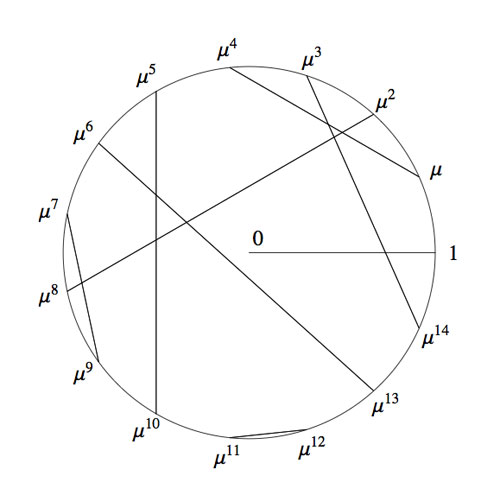Okay, we now have two identifications of the algebraic closure $\overline{\mathbb{F}_2}$ : the smaller ordinals equipped with Nim addition and Nim multiplication and the odd roots of unity with complex-multiplication and the Connes-coupling s. The question we started from asks for a general recipe to identify these two approaches.

To those of you who are convinced that finite fields (LOL, even characteristic two!) are objects far too trivial to bother thinking about : as far as I know, NOBODY knows how to do this explicitly, even restricting the ordinals to merely the natural numbers!

Please feel challenged! To get you started, I’ll show you how to place the first 15 Knights and give you a procedure (though far from explicit) to continue. Here’s the Nim-picture compatible with that above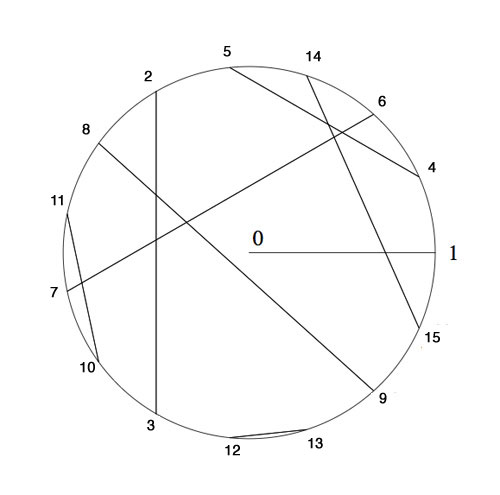To verify this, and to illustrate the general strategy, I’d better hand you the Nim-tables of the first 16 numbers. Here they are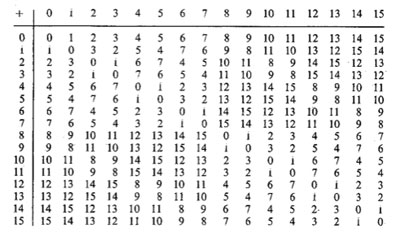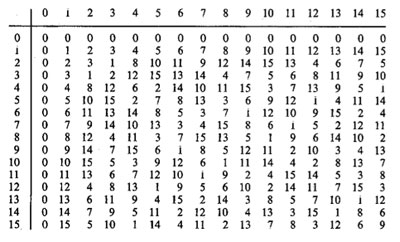It is known that the finite subfields of $~(\mathbb{N},\oplus,\otimes)$ are precisely the sets of numbers smaller than the Fermat-powers $F_n$. So, the first one is all numbers smaller than $F_1=4$ (check!). The smallest generator of the multiplicative group (of order 3) is 2, so we take this to correspond to the unit-root $e^{2 \pi i/3}$. The next subfield are all numbers smaller than $F_2 = 16$ and its multiplicative group has order 15. Now, choose the smallest integer k which generates this group, compatible with the condition that $k^{\otimes 5}=2$. Verify that this number is 4 and that this forces the identification and coupling given above.

The next finite subfield would consist of all natural numbers smaller than $F_3=256$. Hence, in this field we are looking for the smallest number k generating the multiplicative group of order 255 satisfying the extra condition that $k^{\otimes 17}=4$ which would fix an identification at that level. Then, the next level would be all numbers smaller than $F_4=65536$ and again we would like to find the smallest number generating the multiplicative group and such that the appropriate power is equal to the aforementioned k, etc. etc.

Can you give explicit (even inductive) formulae to achieve this? I guess even the problem of placing Knight 16 will give you a couple of hours to think about… (to be continued).

I really like Matilde Marcolli’s idea to use some of Jackson Pollock’s paintings as metaphors for noncommutative spaces. In her talk she used this painting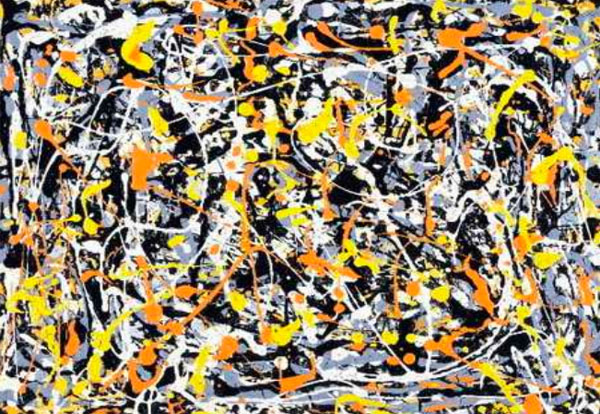and refered to it (as did I in my post) as : Jackson Pollock “Untitled N.3”. Before someone writes a post ‘The Pollock noncommutative space hoax’ (similar to my own post) let me point out that I am well aware of the controversy surrounding this painting.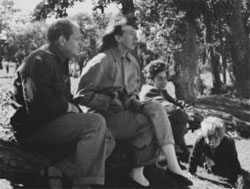This painting is among 32 works recently discovered and initially attributed to Pollock.
In fact, I’ve already told part of the story in Doodles worth millions (or not)? (thanks to PD1). The story involves the people on the right : from left to right, Jackson Pollock, his wife Lee Krasner, Mercedes Matter and her son Alex Matter.

Alex Matter, whose father, Herbert, and mother, Mercedes, were artists and friends of Jackson Pollock, discovered after his mother died a group of small drip paintings in a storage locker in Wainscott, N.Y. which he believed to be authentic Pollocks.

Read the post mentioned above if you want to know how mathematics screwed up his plan, or much better, reed the article Anatomy of the Jackson Pollock controversy by Stephen Litt.

So, perhaps the painting above was not the smartest choice, but we could take any other genuine Pollock ‘drip-painting’, a technique he taught himself towards the end of 1946 to make an image by splashing, pouring, sloshing colors onto the canvas. Typically, such a painting consists of blops of paint, connected via thin drip-lines.

What does this have to do with noncommutative geometry? Well, consider the blops as ‘points’. In commutative geometry, distinct points cannot share tangent information ((technically : a commutative semi-local ring splits as the direct sum of local rings and this does no longer hold for a noncommutative semi-local ring)). In the noncommutative world though, they can!, or if you want to phrase it like this, noncommutative points ‘can talk to each other’. And, that’s what we cherish in those drip-lines.

But then, if two points share common tangent informations, they must be awfully close to each other… so one might imagine these Pollock-lines to be strings holding these points together. Hence, it would make more sense to consider the ‘Pollock-quotient-painting’, that is, the space one gets after dividing out the relation ‘connected by drip-lines’ ((my guess is that Matilde thinks of the lines as the action of a group on the points giving a topological horrible quotient space, and thats precisely where noncommutative geometry shines)).

For this reason, my own mental picture of a genuinely noncommutative space ((that is, the variety corresponding to a huge noncommutative algebra such as free algebras, group algebras of arithmetic groups or fundamental groups)) looks more like the picture below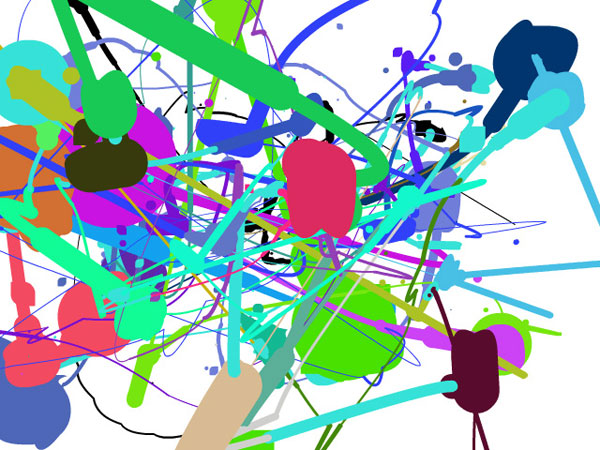The colored blops you see are really sets of points which you might view as, say, a FacebookGroup ((technically, think of them as the connected components of isomorphism classes of finite dimensional simple representations of your favorite noncommutative algebra)). Some chatter may occur between two distinct FacebookGroups, the more chatter the thicker the connection depicted ((technically, the size of the connection is the dimension of the ext-group between generic simples in the components)). Now, there are some tiny isolated spots (say blue ones in the upper right-hand quadrant). These should really be looked at as remote clusters of noncommutative points (sharing no (tangent) information whatsoever with the blops in the foregound). If we would zoom into them beyond the Planck scale (if I’m allowed to say a bollock-word in a Pollock-post) they might reveal again a whole universe similar to the interconnected blops upfront.

The picture was produced using the fabulous Pollock engine. Just use your mouse to draw and click to change colors in order to produce your very own noncommutative space!

For the mathematicians still around, this may sound like a lot of Pollock-bollocks but can be made precise. See my note Noncommutative geometry and dual coalgebras for a very terse reading. Now that coalgebras are gaining popularity, I really should write a more readable account of it, including some fanshi-wanshi examples…

The general public expects pictures from geometers, even from non-commutative geometers. Hence, it is important for researchers in this topic to make an attempt to convey the mental picture they have of their favourite noncommutative space, … somehow. Two examples :This picture was created by Shahn Majid. It appears on his visions of noncommutative geometry page as well as in an extremely readable Plus-magazine article on Quantum geometry, written by Marianne Freiberger, explaining Shahn’s ideas. For more information on this, read Shahn’s SpaceTime blog.This painting is Jackson Pollock‘s “Untitled N.3”. It depicts the way Matilde Marcolli imagines a noncommutative space. It is taken from her slides of her talk for a general audience Mathematicians look at particle physics.Last time we did recall Cantor’s addition and multiplication on ordinal numbers. Note that we can identify an ordinal number $\alpha$ with (the order type of) the set of all strictly smaller ordinals, that is, $\alpha = { \alpha’~:~\alpha’ < \alpha }$. Given two ordinals $\alpha$ and $\beta$ we will denote their Cantor-sums and products as $[ \alpha + \beta]$ and $[\alpha . \beta]$.

The reason for these square brackets is that John Conway constructed a well behaved nim-addition and nim-multiplication on all ordinals $\mathbf{On}_2$ by imposing the ‘simplest’ rules which make $\mathbf{On}_2$ into a field. By this we mean that, in order to define the addition $\alpha + \beta$ we must have constructed before all sums $\alpha’ + \beta$ and $\alpha + \beta’$ with $\alpha’ < \alpha$ and $\beta’ < \beta$. If + is going to be a well-defined addition on $\mathbf{On}_2$ clearly $\alpha + \beta$ cannot be equal to one of these previously constructed sums and the ‘simplicity rule’ asserts that we should take $\alpha+\beta$ the least ordinal different from all these sums $\alpha’+\beta$ and $\alpha+\beta’$. In symbols, we define

$\alpha+ \beta = \mathbf{mex} { \alpha’+\beta,\alpha+ \beta’~|~\alpha’ < \alpha, \beta’ < \beta }$

where $\mathbf{mex}$ stands for ‘minimal excluded value’. If you’d ever played the game of Nim you will recognize this as the Nim-addition, at least when $\alpha$ and $\beta$ are finite ordinals (that is, natural numbers) (to nim-add two numbers n and m write them out in binary digits and add without carrying). Alternatively, the nim-sum n+m can be found applying the following two rules :

• the nim-sum of a number of distinct 2-powers is their ordinary sum (e.g. $8+4+1=13$, and,
• the nim-sum of two equal numbers is 0.

So, all we have to do is to write numbers n and m as sums of two powers, scratch equal terms and add normally. For example, $13+7=(8+4+1)+(4+2+1)=8+2=10$ (of course this is just digital sum without carry in disguise).Here’s the beginning of the nim-addition table on ordinals. For example, to define $13+7$ we have to look at all values in the first 7 entries of the row of 13 (that is, ${ 13,12,15,14,9,8,11 }$) and the first 13 entries in the column of 7 (that is, ${ 7,6,5,4,3,2,1,0,15,14,13,12,11 }$) and find the first number not included in these two sets (which is indeed $10$).

In fact, the above two rules allow us to compute the nim-sum of any two ordinals. Recall from last time that every ordinal can be written uniquely as as a finite sum of (ordinal) 2-powers :
$\alpha = [2^{\alpha_0} + 2^{\alpha_1} + \ldots + 2^{\alpha_k}]$, so to determine the nim-sum $\alpha+\beta$ we write both ordinals as sums of ordinal 2-powers, delete powers appearing twice and take the Cantor ordinal sum of the remaining sum.Nim-multiplication of ordinals is a bit more complicated. Here’s the definition as a minimal excluded value

$\alpha.\beta = \mathbf{mex} { \alpha’.\beta + \alpha.\beta’ – \alpha’.\beta’ }$

for all $\alpha’ < \alpha, \beta’ < \beta$. The rationale behind this being that both $\alpha-\alpha’$ and $\beta – \beta’$ are non-zero elements, so if $\mathbf{On}_2$ is going to be a field under nim-multiplication, their product should be non-zero (and hence strictly greater than 0), that is, $~(\alpha-\alpha’).(\beta-\beta’) > 0$. Rewriting this we get $\alpha.\beta > \alpha’.\beta+\alpha.\beta’-\alpha’.\beta’$ and again the ‘simplicity rule’ asserts that $\alpha.\beta$ should be the least ordinal satisfying all these inequalities, leading to the $\mathbf{mex}$-definition above. The table gives the beginning of the nim-multiplication table for ordinals. For finite ordinals n and m there is a simple 2 line procedure to compute their nim-product, similar to the addition-rules mentioned before :

• the nim-product of a number of distinct Fermat 2-powers (that is, numbers of the form $2^{2^n}$) is their ordinary product (for example, $16.4.2=128$), and,
• the square of a Fermat 2-power is its sesquimultiple (that is, the number obtained by multiplying with $1\frac{1}{2}$ in the ordinary sense). That is, $2^2=3,4^2=6,16^2=24,…$

Using these rules, associativity and distributivity and our addition rules it is now easy to work out the nim-multiplication $n.m$ : write out n and m as sums of (multiplications by 2-powers) of Fermat 2-powers and apply the rules. Here’s an example

$5.9=(4+1).(4.2+1)=4^2.2+4.2+4+1=6.2+8+4+1=(4+2).2+13=4.2+2^2+13=8+3+13=6$

Clearly, we’d love to have a similar procedure to calculate the nim-product $\alpha.\beta$ of arbitrary ordinals, or at least those smaller than $\omega^{\omega^{\omega}}$ (recall that Conway proved that this ordinal is isomorphic to the algebraic closure $\overline{\mathbb{F}}_2$ of the field of two elements). From now on we restrict to such ‘small’ ordinals and we introduce the following special elements :

$\kappa_{2^n} = [2^{2^{n-1}}]$ (these are the Fermat 2-powers) and for all primes $p > 2$ we define
$\kappa_{p^n} = [\omega^{\omega^{k-1}.p^{n-1}}]$ where $k$ is the number of primes strictly smaller than $p$ (that is, for p=3 we have k=1, for p=5, k=2 etc.).

Again by associativity and distributivity we will be able to multiply two ordinals $< \omega^{\omega^{\omega}}$ if we know how to multiply a product

$[\omega^{\alpha}.2^{n_0}].[\omega^{\beta}.2^{m_0}]$ with $\alpha,\beta < [\omega^{\omega}]$ and $n_0,m_0 \in \mathbb{N}$.

Now, $\alpha$ can be written uniquely as $[\omega^t.n_t+\omega^{t-1}.n_{t-1}+\ldots+\omega.n_2 + n_1]$ with t and all $n_i$ natural numbers. Write each $n_k$ in base $p$ where $p$ is the $k+1$-th prime number, that is, we have for $n_0,n_1,\ldots,n_t$ an expression

$n_k=[\sum_j p^j.m(j,k)]$ with $0 \leq m(j,k) < p$

The point of all this is that any of the special elements we want to multiply can be written as a unique expression as a decreasing product

$[\omega^{\alpha}.2^{n_0}] = [ \prod_q \kappa_q^m(q) ]$

where $q$ runs over all prime powers. The crucial fact now is that for this decreasing product we have a rule similar to addition of 2-powers, that is Conway-products coincide with the Cantor-products

$[ \prod_q \kappa_q^m(q) ] = \prod_q \kappa_q^m(q)$

But then, using associativity and commutativity of the Conway-product we can ‘nearly’ describe all products $[\omega^{\alpha}.2^{n_0}].[\omega^{\beta}.2^{m_0}]$. The remaining problem being that it may happen that for some q we will end up with an exponent $m(q)+m(q’)>p$. But this can be solved if we know how to take p-powers. The rules for this are as follows

$~(\kappa_{2^n})^2 = \kappa_{2^n} + \prod_{1 \leq i < n} \kappa_{2^i}$, for 2-powers, and,

$~(\kappa_{p^n})^p = \kappa_{p^{n-1}}$ for a prime $p > 2$ and for $n \geq 2$, and finally

$~(\kappa_p)^p = \alpha_p$ for a prime $p > 2$, where $\alpha_p$ is the smallest ordinal $< \kappa_p$ which cannot be written as a p-power $\beta^p$ with $\beta < \kappa_p$. Summarizing : if we will be able to find these mysterious elements $\alpha_p$ for all prime numbers p, we are able to multiply in $[\omega^{\omega^{\omega}}]=\overline{\mathbb{F}}_2$.

Let us determine the first one. We have that $\kappa_3 = \omega$ so we are looking for the smallest natural number $n < \omega$ which cannot be written in num-multiplication as $n=m^3$ for $m < \omega$ (that is, also $m$ a natural number). Clearly $1=1^3$ but what about 2? Can 2 be a third root of a natural number wrt. nim-multiplication? From the tabel above we see that 2 has order 3 whence its cube root must be an element of order 9. Now, the only finite ordinals that are subfields of $\mathbf{On}_2$ are precisely the Fermat 2-powers, so if there is a finite cube root of 2, it must be contained in one of the finite fields $[2^{2^n}]$ (of which the mutiplicative group has order $2^{2^n}-1$ and one easily shows that 9 cannot be a divisor of any of the numbers $2^{2^n}-1$, that is, 2 doesn’t have a finte 3-th root in nim! Phrased differently, we found our first mystery number $\alpha_3 = 2$. That is, we have the marvelous identity in nim-arithmetic

$\omega^3 = 2$

Okay, so what is $\alpha_5$? Well, we have $\kappa_5 = [\omega^{\omega}]$ and we have to look for the smallest ordinal which cannot be written as a 5-th root. By inspection of the finite nim-table we see that 1,2 and 3 have 5-th roots in $\omega$ but 4 does not! The reason being that 4 has order 15 (check in the finite field ) and 25 cannot divide any number of the form $2^{2^n}-1$. That is, $\alpha_5=4$ giving another crazy nim-identity

$~(\omega^{\omega})^5 = 4$

And, surprises continue to pop up… Conway showed that $\alpha_7 = \omega+1$ giving the nim-identity $~(\omega^{\omega^2})^7 = \omega+1$. The proof of this already uses some clever finite field arguments. Because 7 doesn’t divide any number $2^{2^n}-1$, none of the finite subfields $[2^{2^n}]$ contains a 7-th root of unity, so the 7-power map is injective whence surjective, so all finite ordinal have finite 7-th roots! That is, $\alpha_7 \geq \omega$. Because $\omega$ lies in a cubic extension of the finite field , the field generated by $\omega$ has 64 elements and so its multiplicative group is cyclic of order 63 and as $\omega$ has order 9, it must be a 7-th power in this field. But, as the only 7th powers in that field are precisely the powers of $\omega$ and by inspection $\omega+1$ is not a 7-th power in that field (and hence also not in any field extension obtained by adjoining square, cube and fifth roots) so $\alpha_7=\omega +1$.

Conway did stop at $\alpha_7$ but I’ve always been intrigued by that one line in ONAG p.61 : “Hendrik Lenstra has computed $\alpha_p$ for $p \leq 43$”. Next time we will see how Lenstra managed to do this and we will use sage to extend his list a bit further, including the first open case : $\alpha_{47}= \omega^{\omega^7}+1$.

For an enjoyable video on all of this, see Conway’s MSRI lecture on Infinite Games. The nim-arithmetic part is towards the end of the lecture but watching the whole video is a genuine treat!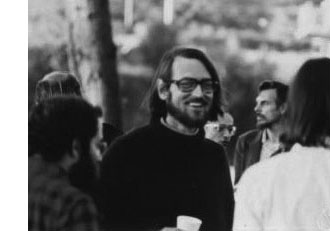David Mumford did receive earlier this year the 2007 AMS Leroy P. Steele Prize for Mathematical Exposition. The jury honors Mumford for “his beautiful expository accounts of a host of aspects of algebraic geometry”. Not surprisingly, the first work they mention are his mimeographed notes of the first 3 chapters of a course in algebraic geometry, usually called “Mumford’s red book” because the notes were wrapped in a red cover. In 1988, the notes were reprinted by Springer-Verlag. Unfortnately, the only red they preserved was in the title.

The AMS describes the importance of the red book as follows. “This is one of the few books that attempt to convey in pictures some of the highly abstract notions that arise in the field of algebraic geometry. In his response upon receiving the prize, Mumford recalled that some of his drawings from The Red Book were included in a collection called Five Centuries of French Mathematics. This seemed fitting, he noted: “After all, it was the French who started impressionist painting and isn’t this just an impressionist scheme for rendering geometry?””

These days it is perfectly possible to get a good grasp on difficult concepts from algebraic geometry by reading blogs, watching YouTube or plugging in equations to sophisticated math-programs. In the early seventies though, if you wanted to know what Grothendieck’s scheme-revolution was all about you had no choice but to wade through the EGA’s and SGA’s and they were notorious for being extremely user-unfriendly regarding illustrations…

So the few depictions of schemes available, drawn by people sufficiently fluent in Grothendieck’s new geometric language had no less than treasure-map-cult-status and were studied in minute detail. Mumford’s red book was a gold mine for such treasure maps. Here’s my favorite one, scanned from the original mimeographed notes (it looks somewhat tidier in the Springer-version)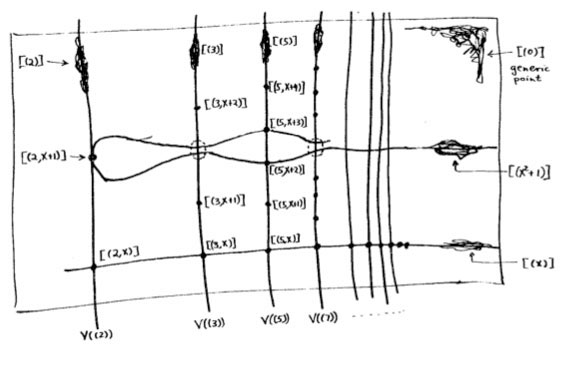It is the first depiction of $\mathbf{spec}(\mathbb{Z}[x])$, the affine scheme of the ring $\mathbb{Z}[x]$ of all integral polynomials. Mumford calls it the”arithmetic surface” as the picture resembles the one he made before of the affine scheme $\mathbf{spec}(\mathbb{C}[x,y])$ corresponding to the two-dimensional complex affine space $\mathbb{A}^2_{\mathbb{C}}$. Mumford adds that the arithmetic surface is ‘the first example which has a real mixing of arithmetic and geometric properties’.

Let’s have a closer look at the treasure map. It introduces some new signs which must have looked exotic at the time, but have since become standard tools to depict algebraic schemes.

For starters, recall that the underlying topological space of $\mathbf{spec}(\mathbb{Z}[x])$ is the set of all prime ideals of the integral polynomial ring $\mathbb{Z}[x]$, so the map tries to list them all as well as their inclusions/intersections.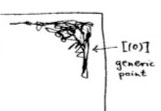The doodle in the right upper corner depicts the ‘generic point’ of the scheme. That is, the geometric object corresponding to the prime ideal $~(0)$ (note that $\mathbb{Z}[x]$ is an integral domain). Because the zero ideal is contained in any other prime ideal, the algebraic/geometric mantra (“inclusions reverse when shifting between algebra and geometry”) asserts that the gemetric object corresponding to $~(0)$ should contain all other geometric objects of the arithmetic plane, so it is just the whole plane! Clearly, it is rather senseless to depict this fact by coloring the whole plane black as then we wouldn’t be able to see the finer objects. Mumford’s solution to this is to draw a hairy ball, which in this case, is sufficiently thick to include fragments going in every possible direction. In general, one should read these doodles as saying that the geometric object represented by this doodle contains all other objects seen elsewhere in the picture if the hairy-ball-doodle includes stuff pointing in the direction of the smaller object. So, in the case of the object corresponding to $~(0)$, the doodle has pointers going everywhere, saying that the geometric object contains all other objects depicted.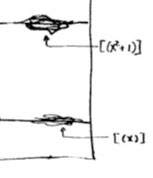Let’s move over to the doodles in the lower right-hand corner. They represent the geometric object corresponding to principal prime ideals of the form $~(p(x))$, where $p(x)$ in an irreducible polynomial over the integers, that is, a polynomial which we cannot write as the product of two smaller integral polynomials. The objects corresponding to such prime ideals should be thought of as ‘horizontal’ curves in the plane.

The doodles depicted correspond to the prime ideal $~(x)$, containing all polynomials divisible by $x$ so when we divide it out we get, as expected, a domain $\mathbb{Z}[x]/(x) \simeq \mathbb{Z}$, and the one corresponding to the ideal $~(x^2+1)$, containing all polynomials divisible by $x^2+1$, which can be proved to be a prime ideals of $\mathbb{Z}[x]$ by observing that after factoring out we get $\mathbb{Z}[x]/(x^2+1) \simeq \mathbb{Z}[i]$, the domain of all Gaussian integers $\mathbb{Z}[i]$. The corresponding doodles (the ‘generic points’ of the curvy-objects) have a predominant horizontal component as they have the express the fact that they depict horizontal curves in the plane. It is no coincidence that the doodle of $~(x^2+1)$ is somewhat bulkier than the one of $~(x)$ as the later one must only depict the fact that all points lying on the straight line to its left belong to it, whereas the former one must claim inclusion of all points lying on the ‘quadric’ it determines.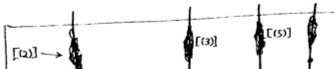Apart from these ‘horizontal’ curves, there are also ‘vertical’ lines corresponding to the principal prime ideals $~(p)$, containing the polynomials, all of which coefficients are divisible by the prime number $p$. These are indeed prime ideals of $\mathbb{Z}[x]$, because their quotients are
$\mathbb{Z}[x]/(p) \simeq (\mathbb{Z}/p\mathbb{Z})[x]$ are domains, being the ring of polynomials over the finite field $\mathbb{Z}/p\mathbb{Z} = \mathbb{F}_p$. The doodles corresponding to these prime ideals have a predominant vertical component (depicting the ‘vertical’ lines) and have a uniform thickness for all prime numbers $p$ as each of them only has to claim ownership of the points lying on the vertical line under them.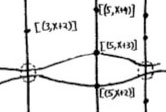Right! So far we managed to depict the zero prime ideal (the whole plane) and the principal prime ideals of $\mathbb{Z}[x]$ (the horizontal curves and the vertical lines). Remains to depict the maximal ideals. These are all known to be of the form
$\mathfrak{m} = (p,f(x))$
where $p$ is a prime number and $f(x)$ is an irreducible integral polynomial, which remains irreducible when reduced modulo $p$ (that is, if we reduce all coefficients of the integral polynomial $f(x)$ modulo $p$ we obtain an irreducible polynomial in $~\mathbb{F}_p[x]$). By the algebra/geometry mantra mentioned before, the geometric object corresponding to such a maximal ideal can be seen as the ‘intersection’ of an horizontal curve (the object corresponding to the principal prime ideal $~(f(x))$) and a vertical line (corresponding to the prime ideal $~(p)$). Because maximal ideals do not contain any other prime ideals, there is no reason to have a doodle associated to $\mathfrak{m}$ and we can just depict it by a “point” in the plane, more precisely the intersection-point of the horizontal curve with the vertical line determined by $\mathfrak{m}=(p,f(x))$. Still, Mumford’s treasure map doesn’t treat all “points” equally. For example, the point corresponding to the maximal ideal $\mathfrak{m}_1 = (3,x+2)$ is depicted by a solid dot $\mathbf{.}$, whereas the point corresponding to the maximal ideal $\mathfrak{m}_2 = (3,x^2+1)$ is represented by a fatter point $\circ$. The distinction between the two ‘points’ becomes evident when we look at the corresponding quotients (which we know have to be fields). We have

$\mathbb{Z}[x]/\mathfrak{m}_1 = \mathbb{Z}[x]/(3,x+2)=(\mathbb{Z}/3\mathbb{Z})[x]/(x+2) = \mathbb{Z}/3\mathbb{Z} = \mathbb{F}_3$ whereas $\mathbb{Z}[x]/\mathfrak{m}_2 = \mathbb{Z}[x]/(3,x^2+1) = \mathbb{Z}/3\mathbb{Z}[x]/(x^2+1) = \mathbb{F}_3[x]/(x^2+1) = \mathbb{F}_{3^2}$

because the polynomial $x^2+1$ remains irreducible over $\mathbb{F}_3$, the quotient $\mathbb{F}_3[x]/(x^2+1)$ is no longer the prime-field $\mathbb{F}_3$ but a quadratic field extension of it, that is, the finite field consisting of 9 elements $\mathbb{F}_{3^2}$. That is, we represent the ‘points’ lying on the vertical line corresponding to the principal prime ideal $~(p)$ by a solid dot . when their quotient (aka residue field is the prime field $~\mathbb{F}_p$, by a bigger point $\circ$ when its residue field is the finite field $~\mathbb{F}_{p^2}$, by an even fatter point $\bigcirc$ when its residue field is $~\mathbb{F}_{p^3}$ and so on, and on. The larger the residue field, the ‘fatter’ the corresponding point.

In fact, the ‘fat-point’ signs in Mumford’s treasure map are an attempt to depict the fact that an affine scheme contains a lot more information than just the set of all prime ideals. In fact, an affine scheme determines (and is determined by) a “functor of points”. That is, to every field (or even every commutative ring) the affine scheme assigns the set of its ‘points’ defined over that field (or ring). For example, the $~\mathbb{F}_p$-points of $\mathbf{spec}(\mathbb{Z}[x])$ are the solid . points on the vertical line $~(p)$, the $~\mathbb{F}_{p^2}$-points of $\mathbf{spec}(\mathbb{Z}[x])$ are the solid . points and the slightly bigger $\circ$ points on that vertical line, and so on.

This concludes our first attempt to decypher Mumford’s drawing, but if we delve a bit deeper, we are bound to find even more treasures… (to be continued).

A quick recap of last time. We are trying to make sense of affine varieties over the elusive field with one element $\mathbb{F}_1$, which by Grothendieck’s scheme-philosophy should determine a functor

$\mathbf{nano}(N)~:~\mathbf{abelian} \rightarrow \mathbf{sets} \qquad A \mapsto N(A)$

from finite Abelian groups to sets, typically giving pretty small sets $N(A)$. Using the F_un mantra that $\mathbb{Z}$ should be an algebra over $\mathbb{F}_1$ any $\mathbb{F}_1$-variety determines an integral scheme by extension of scalars, as well as a complex variety (by extending further to $\mathbb{C}$). We have already connected the complex variety with the original functor into a gadget that is a couple $~(\mathbf{nano}(N),\mathbf{maxi}(R))$ where $R$ is the coordinate ring of a complex affine variety $X_R$ having the property that every element of $N(A)$ can be realized as a $\mathbb{C} A$-point of $X_R$. Ringtheoretically this simply means that to every element $x \in N(A)$ there is an algebra map $N_x~:~R \rightarrow \mathbb{C} A$.

Today we will determine which gadgets determine an integral scheme, and do so uniquely, and call them the sought for affine schemes over $\mathbb{F}_1$.

Let’s begin with our example : $\mathbf{nano}(N) = \underline{\mathbb{G}}_m$ being the forgetful functor, that is $N(A)=A$ for every finite Abelian group, then the complex algebra $R= \mathbb{C}[x,x^{-1}]$ partners up to form a gadget because to every element $a \in N(A)=A$ there is a natural algebra map $N_a~:~\mathbb{C}[x,x^{-1}] \rightarrow \mathbb{C} A$ defined by sending $x \mapsto e_a$. Clearly, there is an obvious integral form of this complex algebra, namely $\mathbb{Z}[x,x^{-1}]$ but we have already seen that this algebra represents the mini-functor

$\mathbf{min}(\mathbb{Z}[x,x^{-1}])~:~\mathbf{abelian} \rightarrow \mathbf{sets} \qquad A \mapsto (\mathbb{Z} A)^*$

and that the group of units $(\mathbb{Z} A)^*$ of the integral group ring $\mathbb{Z} A$ usually is a lot bigger than $N(A)=A$. So, perhaps there is another less obvious $\mathbb{Z}$-algebra $S$ doing a much better job at approximating $N$? That is, if we can formulate this more precisely…

In general, every $\mathbb{Z}$-algebra $S$ defines a gadget $\mathbf{gadget}(S) = (\mathbf{mini}(S),\mathbf{maxi}(S \otimes_{\mathbb{Z}} \mathbb{C}))$ with the obvious (that is, extension of scalars) evaluation map

$\mathbf{mini}(S)(A) = Hom_{\mathbb{Z}-alg}(S, \mathbb{Z} A) \rightarrow Hom_{\mathbb{C}-alg}(S \otimes_{\mathbb{Z}} \mathbb{C}, \mathbb{C} A) = \mathbf{maxi}(S \otimes_{\mathbb{Z}} \mathbb{C})(A)$

Right, so how might one express the fact that the integral affine scheme $X_T$ with integral algebra $T$ is the ‘best’ integral approximation of a gadget $~(\mathbf{nano}(N),\mathbf{maxi}(R))$. Well, to begin its representing functor should at least contain the information given by $N$, that is, $\mathbf{nano}(N)$ is a sub-functor of $\mathbf{mini}(T)$ (meaning that for every finite Abelian group $A$ we have a natural inclusion $N(A) \subset Hom_{\mathbb{Z}-alg}(T, \mathbb{Z} A)$). As to the “best”-part, we must express that all other candidates factor through $T$. That is, suppose we have an integral algebra $S$ and a morphism of gadgets (as defined last time)

$f~:~(\mathbf{nano}(N),\mathbf{maxi}(R)) \rightarrow \mathbf{gadget}(S) = (\mathbf{mini}(S),\mathbf{maxi}(S \otimes_{\mathbb{Z}} \mathbb{C}))$

then there ought to be $\mathbb{Z}$-algebra morphism $T \rightarrow S$ such that the above map $f$ factors through an induced gadget-map $\mathbf{gadget}(T) \rightarrow \mathbf{gadget}(S)$.

Fine, but is this definition good enough in our trivial example? In other words, is the “obvious” integral ring $\mathbb{Z}[x,x^{-1}]$ the best integral choice for approximating the forgetful functor $N=\underline{\mathbb{G}}_m$? Well, take any finitely generated integral algebra $S$, then saying that there is a morphism of gadgets from $~(\underline{\mathbb{G}}_m,\mathbf{maxi}(\mathbb{C}[x,x^{-1}])$ to $\mathbf{gadget}(S)$ means that there is a $\mathbb{C}$-algebra map $\psi~:~S \otimes_{\mathbb{Z}} \mathbb{C} \rightarrow \mathbb{C}[x,x^{-1}]$ such that for every finite Abelian group $A$ we have a commuting diagram

$\xymatrix{A \ar[rr] \ar[d]_e & & Hom_{\mathbb{Z}-alg}(S, \mathbb{Z} A) \ar[d] \\ Hom_{\mathbb{C}-alg}(\mathbb{C}[x,x^{-1}],\mathbb{C} A) \ar[rr]^{- \circ \psi} & & Hom_{\mathbb{C}-alg}(S \otimes_{\mathbb{Z}} \mathbb{C}, \mathbb{C} A)}$

Here, $e$ is the natural evaluation map defined before sending a group-element $a \in A$ to the algebra map defined by $x \mapsto e_a$ and the vertical map on the right-hand side is extensions by scalars. From this data we must be able to show that the image of the algebra map

$\xymatrix{S \ar[r]^{i} & S \otimes_{\mathbb{Z}} \mathbb{C} \ar[r]^{\psi} & \mathbb{C}[x,x^{-1}]}$

is contained in the integral subalgebra $\mathbb{Z}[x,x^{-1}]$. So, take any generator $z$ of $S$ then its image $\psi(z) \in \mathbb{C}[x,x^{-1}]$ is a Laurent polynomial of degree say $d$ (that is, $\psi(z) = c_{-d} x^{-d} + \ldots c_{-1} x^{-1} + c_0 + c_1 x + \ldots + c_d x^d$ with all coefficients a priori in $\mathbb{C}$ and we need to talk them into $\mathbb{Z}$).

Now comes the basic trick : take a cyclic group $A=C_N$ of order $N > d$, then the above commuting diagram applied to the generator of $C_N$ (the evaluation of which is the natural projection map $\pi~:~\mathbb{C}[x.x^{-1}] \rightarrow \mathbb{C}[x,x^{-1}]/(x^N-1) = \mathbb{C} C_N$) gives us the commuting diagram

$\xymatrix{S \ar[r] \ar[d] & S \otimes_{\mathbb{Z}} \mathbb{C} \ar[r]^{\psi} & \mathbb{C}[x,x^{-1}] \ar[d]^{\pi} \\ \mathbb{Z} C_n = \frac{\mathbb{Z}[x,x^{-1}]}{(x^N-1)} \ar[rr]^j & & \frac{\mathbb{C}[x,x^{-1}]}{(x^N-1)}}$

where the horizontal map $j$ is the natural inclusion map. Tracing $z \in S$ along the diagram we see that indeed all coefficients of $\psi(z)$ have to be integers! Applying the same argument to the other generators of $S$ (possibly for varying values of N) we see that , indeed, $\psi(S) \subset \mathbb{Z}[x,x^{-1}]$ and hence that $\mathbb{Z}[x,x^{-1}]$ is the best integral approximation for $\underline{\mathbb{G}}_m$.

That is, we have our first example of an affine variety over the field with one element $\mathbb{F}_1$ : $~(\underline{\mathbb{G}}_m,\mathbf{maxi}(\mathbb{C}[x,x^{-1}]) \rightarrow \mathbf{gadget}(\mathbb{Z}[x,x^{-1}])$.

What makes this example work is that the infinite group $\mathbb{Z}$ (of which the complex group-algebra is the algebra $\mathbb{C}[x,x^{-1}]$) has enough finite Abelian group-quotients. In other words, $\mathbb{F}_1$ doesn’t see $\mathbb{Z}$ but rather its profinite completion $\hat{\mathbb{Z}} = \underset{\leftarrow} \mathbb{Z}/N\mathbb{Z}$… (to be continued when we’ll consider noncommutative $\mathbb{F}_1$-schemes)

In general, an affine $\mathbb{F}_1$-scheme is a gadget with morphism of gadgets
$~(\mathbf{nano}(N),\mathbf{maxi}(R)) \rightarrow \mathbf{gadget}(S)$ provided that the integral algebra $S$ is the best integral approximation in the sense made explicit before. This rounds up our first attempt to understand the Connes-Consani approach to define geometry over $\mathbb{F}_1$ apart from one important omission : we have only considered functors to $\mathbf{sets}$, whereas it is crucial in the Connes-Consani paper to consider more generally functors to graded sets. In the final part of this series we’ll explain what that’s all about.

Last time we have seen how an affine $\mathbb{C}$-algebra R gives us a maxi-functor (because the associated sets are typically huge)

$\mathbf{maxi}(R)~:~\mathbf{abelian} \rightarrow \mathbf{sets} \qquad A \mapsto Hom_{\mathbb{C}-alg}(R, \mathbb{C} A)$

Substantially smaller sets are produced from finitely generated $\mathbb{Z}$-algebras S (therefore called mini-functors)

$\mathbf{mini}(S)~:~\mathbf{abelian} \rightarrow \mathbf{sets} \qquad A \mapsto Hom_{\mathbb{Z}-alg}(S, \mathbb{Z} A)$

Both these functors are ‘represented’ by existing geometrical objects, for a maxi-functor by the complex affine variety $X_R = \mathbf{max}(R)$ (the set of maximal ideals of the algebra R) with complex coordinate ring R and for a mini-functor by the integral affine scheme $X_S = \mathbf{spec}(S)$ (the set of all prime ideals of the algebra S).

The ‘philosophy’ of F_un mathematics is that an object over this virtual field with one element $\mathbb{F}_1$ records the essence of possibly complicated complex- or integral- objects in a small combinatorial thing.

For example, an n-dimensional complex vectorspace $\mathbb{C}^{n}$ has as its integral form a lattice of rank n $\mathbb{Z}^{\oplus n}$. The corresponding $\mathbb{F}_1$-objects only records the dimension n, so it is a finite set consisting of n elements (think of them as the set of base-vectors of the vectorspace).

Similarly, all base-changes of the complex vectorspace $\mathbb{C}^n$ are given by invertible matrices with complex coefficients $GL_n(\mathbb{C})$. Of these base-changes, the only ones leaving the integral lattice $\mathbb{Z}^{\oplus n}$ intact are the matrices having all their entries integers and their determinant equal to $\pm 1$, that is the group $GL_n(\mathbb{Z})$. Of these integral matrices, the only ones that shuffle the base-vectors around are the permutation matrices, that is the group $S_n$ of all possible ways to permute the n base-vectors. In fact, this example also illustrates Tits’ original motivation to introduce $\mathbb{F}_1$ : the finite group $S_n$ is the Weyl-group of the complex Lie group $GL_n(\mathbb{C})$.

So, we expect a geometric $\mathbb{F}_1$-object to determine a much smaller functor from finite abelian groups to sets, and, therefore we call it a nano-functor

$\mathbf{nano}(N)~:~\mathbf{abelian} \rightarrow \mathbf{sets} \qquad A \mapsto N(A)$

but as we do not know yet what the correct geometric object might be we will only assume for the moment that it is a subfunctor of some mini-functor $\mathbf{mini}(S)$. That is, for every finite abelian group A we have an inclusion of sets $N(A) \subset Hom_{\mathbb{Z}-alg}(S,\mathbb{Z} A)$ in such a way that these inclusions are compatible with morphisms. Again, take pen and paper and you are bound to discover the correct definition of what is called a natural transformation, that is, a ‘map’ between the two functors $\mathbf{nano}(N) \rightarrow \mathbf{mini}(S)$.

Right, now to make sense of our virtual F_un geometrical object $\mathbf{nano}(N)$ we have to connect it to properly existing complex- and/or integral-geometrical objects.

Let us define a gadget to be a couple $~(\mathbf{nano}(N),\mathbf{maxi}(R))$ consisting of a nano- and a maxi-functor together with a ‘map’ (that is, a natural transformation) between them

$e~:~\mathbf{nano}(N) \rightarrow \mathbf{maxi}(R)$

The idea of this map is that it visualizes the elements of the set $N(A)$ as $\mathbb{C} A$-points of the complex variety $X_R$ (that is, as a collection of $o(A)$ points of $X_R$, where $o(A)$ is the number of elements of $A$).

In the example we used last time (the forgetful functor) with $N(A)=A$ any group-element $a \in A$ is mapped to the algebra map $\mathbb{C}[x,x^{-1}] \rightarrow \mathbb{C} A~,~x \mapsto e_a$ in $\mathbf{maxi}(\mathbb{C}[x,x^{-1}])$. On the geometry side, the points of the variety associated to $\mathbb{C} A$ are all algebra maps $\mathbb{C} A \rightarrow \mathbb{C}$, that is, the $o(A)$ characters ${ \chi_1,\ldots,\chi_{o(A)} }$. Therefore, a group-element $a \in A$ is mapped to the $\mathbb{C} A$-point of the complex variety $\mathbb{C}^* = X_{\mathbb{C}[x,x^{-1}]}$ consisting of all character-values at $a$ : ${ \chi_1(a),\ldots,\chi_{o(A)}(g) }$.

In mathematics we do not merely consider objects (such as the gadgets defined just now), but also the morphisms between these objects. So, what might be a morphism between two gadgets

$~(\mathbf{nano}(N),\mathbf{maxi}(R)) \rightarrow (\mathbf{nano}(N’),\mathbf{maxi}(R’))$

Well, naturally it should be a ‘map’ (that is, a natural transformation) between the nano-functors $\phi~:~\mathbf{nano}(N) \rightarrow \mathbf{nano}(N’)$ together with a morphism between the complex varieties $X_R \rightarrow X_{R’}$ (or equivalently, an algebra morphism $\psi~:~R’ \rightarrow R$) such that the extra gadget-structure (the evaluation maps) are preserved.

That is, for every finite Abelian group $A$ we should have a commuting diagram of maps

$\xymatrix{N(A) \ar[rr]^{\phi(A)} \ar[d]^{e_N(A)} & & N'(A) \ar[d]^{e_{N’}(A)} \\ Hom_{\mathbb{C}-alg}(R,\mathbb{C} A) \ar[rr]^{- \circ \psi} & & Hom_{\mathbb{C}-alg}(R’,\mathbb{C} A)}$

Not every gadget is a F_un variety though, for those should also have an integral form, that is, define a mini-functor. In fact, as we will see next time, an affine $\mathbb{F}_1$-variety is a gadget determining a unique mini-functor $\mathbf{mini}(S)$.